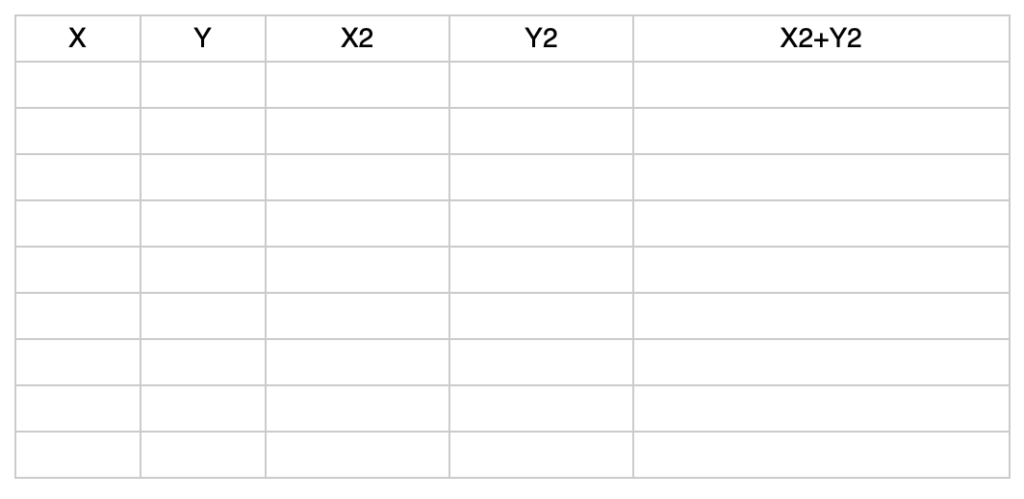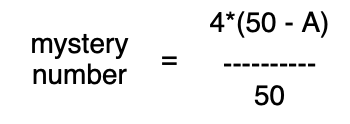# Monte Carlo Method

Get a local phone book (white pages). Pick two adjacent columns on any page. Label the first column with an X and the second column with Y. Pick any phone number in column X. On a separate piece of paper (or in a spreadsheet) write down the last four digits of the phone number and put a decimal point in front of it. For example, if the phone number is 706 555-1234, then you will write .1234 in column X of your piece of paper. Go to the next phone number in the phone book and repeat the process until you have 50 numbers. Do the same for column Y. Find X2, Y2, and X2 + Y2 and make a table similar to the one below:Count how many times the last column is greater than 1 – call this number A. Then calculate the following:Repeat the above procedures until you have 100, 150, 200 data sets, replacing the 50 with the appropriate number in the mystery number calculation.

What is the mystery number? What happens to the calculated value of the mystery number when the number of data increases? Why is the number 4 in the formula for calculating the mystery number?